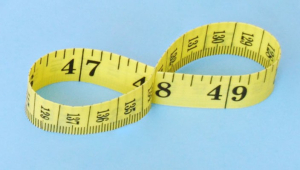# Two mathematicians have proved that two different infinities are equal in size, settling a long-standing question

Kevin Hartnett in Quanta:In a breakthrough that disproves decades of conventional wisdom, two mathematicians have shown that two different variants of infinity are actually the same size. The advance touches on one of the most famous and intractable problems in mathematics: whether there exist infinities between the infinite size of the natural numbers and the larger infinite size of the real numbers.

The problem was first identified over a century ago. At the time, mathematicians knew that “the real numbers are bigger than the natural numbers, but not how much bigger. Is it the next biggest size, or is there a size in between?” said Maryanthe Malliaris of the University of Chicago, co-author of the new work along with Saharon Shelah of the Hebrew University of Jerusalem and Rutgers University.

In their new work, Malliaris and Shelah resolve a related 70-year-old question about whether one infinity (call it p) is smaller than another infinity (call it t). They proved the two are in fact equal, much to the surprise of mathematicians.

More here.